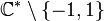# Element structure of special linear group:SL(2,C)

## Contents

View element structure of particular groups | View other specific information about special linear group:SL(2,C)

## Conjugacy class structure

The case of$\mathbb{C}$ is easy because in this case, the characteristic is not two and the field is algebraically closed, which means in particular that every element is a square. We thus do not have to worry about conjugacy classes that can be diagonalized over extensions but not over the field. Also, there is no splitting of conjugacy classes in$SL(2,\mathbb{C})$ relative to$GL(2,\mathbb{C})$, again because every element is a square. In alternative mathematical jargon,$SL(2,\mathbb{C})$ is a central factor (the other factor being the scalar matrices) and hence a conjugacy-closed subgroup of$GL(2,\mathbb{C})$.

Nature of conjugacy class Eigenvalues Characteristic polynomial Minimal polynomial What set can each conjugacy class be identified with? (rough measure of size of conjugacy class) What can the set of conjuacy classes be identified with (rough measure of number of conjugacy classes) What can the union of conjugacy classes be identified with? Semisimple? Diagonalizable over$K$? Splits in$SL_2$ relative to$GL_2$?
Diagonalizable over$\mathbb{C}$ with equal diagonal entries, hence a scalar$\{ 1,1 \}$ or$\{ -1,-1\}$$(x - a)^2$ where$a \in \{ -1,1 \}$$x - a$ where$a \in \{ -1,1\}$ one-point set two-point set (characteristic not two)
one-point set (characteristic two)
two-point set (characteristic not two)
one-point set (characteristic two)
Yes Yes No
Not diagonal, has Jordan block of size two$1$ (multiplicity 2) or$-1$ (multiplicity 2)$(x - a)^2$ where$a \in \{ -1,1 \}$ Same as characteristic polynomial  ?  ?  ? No No No
Diagonalizable over$\mathbb{C}$ with distinct (and hence mutually inverse) diagonal entries$\lambda, 1/\lambda$ where$\lambda \in \mathbb{C} \setminus \{ 0,1,-1 \}$$x^2 - (\lambda + 1/\lambda)x + 1$ Same as characteristic polynomial  ? quotient space of$\mathbb{C}^* \setminus \{ -1,1 \}$ under the inverse map action  ? Yes Yes No

### Identification between conjugacy classes and field elements

The trace is a continuous mapping:

Conjugacy classes in$SL(2,\mathbb{C})$$\to$$\mathbb{C}$

Further, this mapping is almost bijective. For any$a \ne -2,2$, there is a unique conjugacy class in$SL(2,\mathbb{C})$ with trace$a$. For$a \in \{ -2,2 \}$, there are two conjugacy classes mapping to$a$: the scalar matrix class and the Jordan block class.

If we give the set of conjugacy classes in$SL(2,\mathbb{C})$ the quotient topology from the topology on$SL(2,\mathbb{C})$, it will look as follows: setwise, it is a union of$\mathbb{C}$ and two open points. One of the open points has closure defined to be that point and$-2 \in \mathbb{C}$. The other open point has closure defined to be that point and$2 \in \mathbb{C}$. The open points correspond to the Jordan block classes and the points -2,2 in$\mathbb{C}$ to the scalar matrix classes.